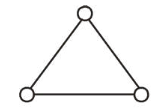# Consider a gas of triatomic molecules.Question:Consider a gas of triatomic molecules. The molecules are assumed to be triangular and made of massless rigid rods whose vertices are occupied by atoms. The internal energy of a mole of the gas at temperature $T$ is :

1. (1) $\frac{5}{2} R T$

2. (2) $\frac{3}{2} R T$

3. (3) $\frac{9}{2} R T$

4. (4) $3 R T$

Correct Option: 4,

Solution:

(4) Here degree of freedom, $f=3+3=6$ for triatomic nonlinear molecule.

Internal energy of a mole of the gas at temperature $T$,

$U=\frac{f}{2} n R T=\frac{6}{2} R T=3 R T$